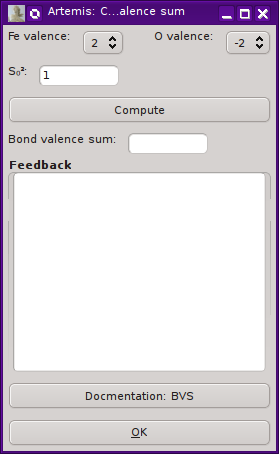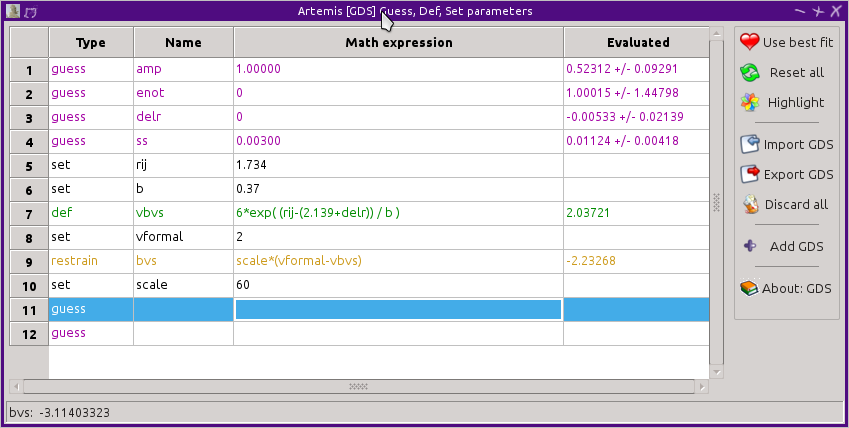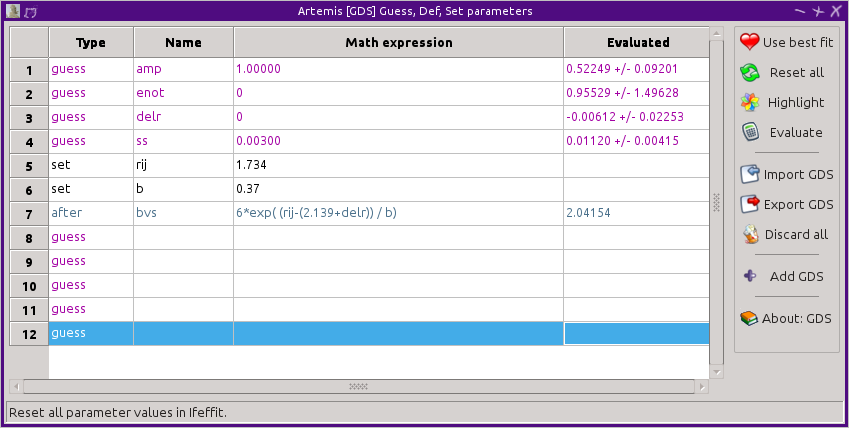# 15.6. Bond valence sums¶

The concept of a bond in inorganic or crystal chemistry is a bit ambiguous. In two seminal pair of papers, Brown and Altermatt proposed this definition “All neighboring cation-anion distances are considered to be bonds although not all of equal strength.” In this model, each bond between atoms i and j has a number – the bond valence – sij which is inversely proportional to bond distance. The bond valence is defined as sij= exp((R0,ij - Rij)/B), where Rij is the contact distance and R0,ij and B are empirically determined parameters.

• D. Altermatt and I. D. Brown. The automatic searching for chemical bonds in inorganic crystal structures. Acta Crystallographica Section B, 41(4):240–244, Aug 1985. doi:10.1107/S0108768185002051.
• I. D. Brown and D. Altermatt. Bond-valence parameters obtained from a systematic analysis of the Inorganic Crystal Structure Database. Acta Crystallographica Section B, 41(4):244–247, Aug 1985. doi:10.1107/S0108768185002063.

They searched through the Inorganic Crystal Structures Database to determine empirical values for sij for over 150 cation/anion pairs. Other authors have supplemented this work with additional anion/cation pairs. Most of these are for common cations, such as oxygen, nitrogen, or sulfur. Interestingly, B is nearly constant across all bonds and equal to 0.37. For some anions such as K and U, the value of B can be as high as about 0.6. R0,ij depends on the contact pair and has been tabulated along with B in a database.

The bond valance sum, then, is the sum of sij over all pairs in a coordination shell: V= Σsij. The bond valence sum V should be equal to the formal valence of the absorber cation. This provides a way of relating coordination number, bond distance, and formal valence in a way that is useful and directly applicable to EXAFS analysis.

## 15.6.1. Computing a bond valence sum from a fit¶

ARTEMIS provides a tool for computing a bond valence sum from a list of paths included in a fit. A set of paths to be included in the sum can be marked in a path list. Selecting Actions ‣ Compute bond valence sum will display this dialog.Fig. 15.16 The bond valence sum dialog.

Some care is taken to verify that your selection of paths is sensible. ARTEMIS will notice if you have marked multiple scattering paths or have marked paths with absorber/scatterer pairs that are not in the bond valence database. Although it will proceed with a calculation, ARTEMIS will warn you if it seems as though you have included paths that do not seem to be a part of the first coordination shell.

ARTEMIS also tries to make good guesses about the formal valences of the absorber and scatterer, althoguh it will often guess wrongly. It is, therefore, essential that you set the valences correctly using the choice menus at the top of the bond valence dialog. It is much more likely that the absorber valence will be guessed incorrectly.

You will notice that one of the valence options for many absorber species is “9”, an obviously wrong value of valence. The bond valence database says “Bond valence parameters for atoms whose oxidation state is given as 9 do not have an oxidation state specified in the original citation. They may apply to a particular, but unspecified, oxidation state or they may be intended to apply to all oxidation states.”

In order to make the bond valence summation, the degeneracy of each path included in the sum must be multiplied by its evaluation of sij (which also uses the evaluation of R=R0+ ΔR as the value of Rij). Because path degeneracy might need to consider quite complicated parameterization of the S02 path parameter as well as the N path parameter, ARTEMIS will multiply the evaluations of the N and S02 path parameters together to use as the evaluation of degeneracy in the summmation. It is up to you, the user, to supply a value for the actual amplitude reduction factor, S02 to be divided out of the summation.

Pressing the Compute button will make the bond valence sum, reporting its value in the text box. Any feedback will be written in the larger text control. For a successful calculation, the values of Rij and B obtained from the database will be displayed. Any warnings about the path selection will be printed in the feedback box in bold red text.

## 15.6.2. Using a bond valance sum as a restraint¶

The bond valence sum can be used a restraint on a fit. That is, the relationship between formal valence, coordination number, and bond distance can be used as prior knowledge guiding the fit. If the absorber/scatterer pair are in the bond valence database, values for R0,ij, B, and the formal valence of the obsorber can be defined as set parameters. The bond valence sum is expressed as a def parameter. Finally, the difference between the bond valence sum and the formal valence are expressed as a restrain parameter. These are shown below for the Fe-O bond in FeO. In FeO the iron atom is of valence 2+ and the oxygen is 2-.Fig. 15.17 Defining a group of parameters to make a restraint based on a bond valence sum.

When the fit is evaluated, the restrain parameter will be added in quadrature to the evaluation of χ2. This sum will be minimized in the fit. In a fit to FeO, the coordination number is fixed to 6, the value known from crystallography. By using this restraint, the value of ΔR will be encouraged to assume a value that results in a bond valence sum of 2. By increasing the value of the scale parameter, the strength of the restraint is increased. For a very large value of scale, ΔR will constrained to a value that forces the bond valence sum to 2. For a very small value of scale, the restraint will be weak and ΔR will be given more freedom to deviate from a value that casues a bond valence sum of 2.

This example shows the simplest case of a single scattering path contributing to the bond valence sum. The math expressions to establish the restraint would be more complicated for a more disrodered first shell, but those math expressions would follow the same pattern as this example.

## 15.6.3. Using a bond valance sum as an after parameter¶

The last ARTEMIS trick related to evaluations of bond valence sums is to use an after parameter to record the bond valence sum to the log file. Using the same set of parameters as in the restraint example, set the BVS formula instead to an after parameter.Fig. 15.18 Defining an after parameter which reports the evaluated bond valence sum to the log file.

At the end of the fit, the BVS will be evaluated and reported in the log file just below the guess, def, and set parameters, like so:

```after parameters:
bvs                =   2.04154071    # [6*exp( (rij-(2.139+delr)) / b)]
```